International
Tables for
Crystallography
Volume B
Reciprocal space
Edited by U. Shmueli

International Tables for Crystallography (2006). Vol. B. ch. 2.1, pp. 190-191   | 1 | 2 |

## Section 2.1.2.1. Mathematical background

U. Shmuelia* and A. J. C. Wilsonb

aSchool of Chemistry, Tel Aviv University, Tel Aviv 69 978, Israel, and bSt John's College, Cambridge, England
Correspondence e-mail:  ushmueli@post.tau.ac.il

#### 2.1.2.1. Mathematical background

| top | pdf |

The process may be illustrated by evaluating, or attempting to evaluate, the average intensity of reflection by the three processes. The intensity of reflection is given by multiplying equation (2.1.1.1)by its complex conjugate: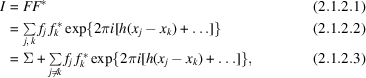where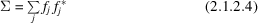is the sum of the squares of the moduli of the atomic scattering factors. Wilson (1942) argued, without detailed calculation, that the average value of the exponential term would be zero and hence that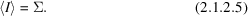Averaging equation (2.1.2.3)forfixed,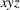ranging uniformly over the unit cell – the first process described above – gives this result identically, without complication or approximation. Ordinarily the second process cannot be carried out. We can, however, postulate a special case in which it is possible. We take a homoatomic structure and before averaging we correct the f's for temperature effects and the fall-off with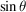, so that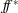is the same for all the atoms and is independent of. If the range ofover which the expression for I has to be averaged is taken as a parallelepiped in reciprocal space with h ranging from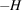to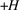, k from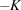to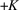, l from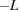to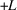, equation (2.1.2.2)can be factorized into the product of the sums of three geometrical progressions. Algebraic manipulation then easily leads to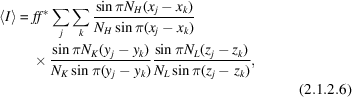where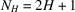,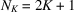and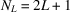. The terms with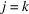give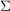, but the remaining terms are not zero. Because of the periodic nature of the trigonometric terms, the effective coordinate differences are never greater than 0.5 and in a structure of any complexity there will be many much less than 0.5. For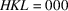, in fact,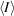becomes the square of the modulus of the sum of the atomic scattering factors,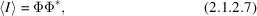where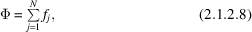and not the sum of the squares of their moduli; for larger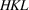,rapidly decreases toand then oscillates about that value. Wilson (1949, especially Section 2.1.1) suggested that the regions of averaging should be chosen so that at least one index of every reflection is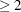ifis to be identified with, and this has proven to be a useful rule-of-thumb.

The third process of averaging replaces the sum over integral values of the indices by an integration over continuous values, the appropriate values of the limits in this example being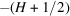to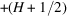. The effect is to replace the sines in the denominators, but not in the numerators, of equation (2.1.2.6)by their arguments, and this is equivalent to the approximation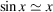in the denominators only. This is a good approximation for atoms close together in the structure and thus giving the largest terms in the sums in equation (2.1.2.6), and gives the correct sign and order of magnitude even for x having its maximum value of.

### ReferencesWilson, A. J. C. (1942). Determination of absolute from relative intensity data. Nature (London), 150, 151–152.Google ScholarWilson, A. J. C. (1949). The probability distribution of X-ray intensities. Acta Cryst. 2, 318–320.Google Scholar